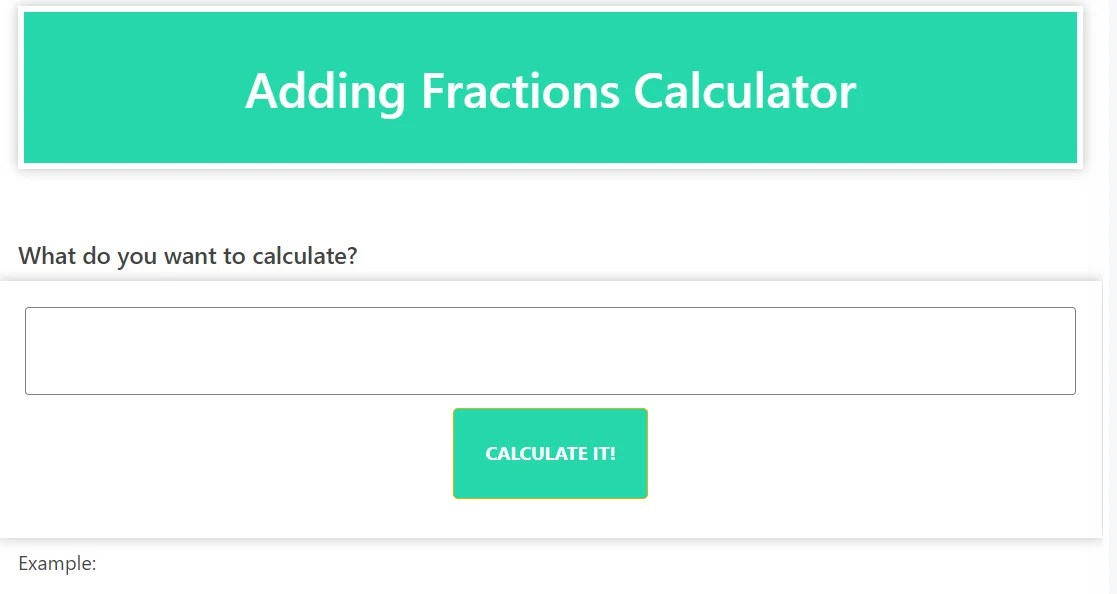# Adding Fractions Calculator (Maths Solver): Unlocking the Simplicity of Fraction Addition

The Adding Fractions Calculator, also known as the Maths Solver, is a powerful tool designed to simplify the process of adding fractions. Whether you’re a student learning fractions, a professional working with fractional values, or an enthusiast in need of quick and accurate solutions, our Adding Fractions Calculator is here to assist you.

Adding fractions manually can be challenging and time-consuming. Our Adding Fractions Calculator eliminates the complexity by automating the addition process. Simply input the fractions you want to add, and our calculator will provide the sum in the simplest form. It handles both proper fractions and improper fractions seamlessly.

The Adding Fractions Calculator can handle a wide range of fractions, including like fractions (fractions with the same denominator) and unlike fractions (fractions with different denominators). It accommodates whole numbers, mixed numbers, and fractions with varying numerators and denominators. Regardless of the complexity or the number of fractions involved, our calculator computes the sum effortlessly.

### Step-by-Step Fraction Solutions:

Understanding the process of adding fractions is crucial for mastering the concept. Our Adding Fractions Calculator not only provides the final sum but also breaks down the steps involved in the addition process. This allows you to follow along and gain a deeper understanding of fraction addition principles.

### Efficiency and Accuracy:

Time is valuable, and our Adding Fractions Calculator ensures both efficiency and accuracy. Automating the addition process saves you valuable time and reduces the risk of calculation errors. Rest assured that the calculator provides precise and reliable results, giving you confidence in the accuracy of the sum.

### Unlock the Power of the Adding Fractions Calculator:

Whether you’re a student studying fractions, a professional working with fractional values, or an enthusiast exploring the world of mathematics, our Adding Fractions Calculator is a valuable resource. Simplify your fraction addition tasks and unlock new possibilities in mathematical problem-solving.

Experience the Advantage of the Adding Fractions Calculator: Transform your approach to fraction addition with our advanced Adding Fractions Calculator. Bid farewell to manual calculations and embrace the efficiency and accuracy of our automated tool. Empower yourself with a calculator that simplifies complex fraction addition. Explore the possibilities with our Adding Fractions Calculator and enhance your mathematical skills today.